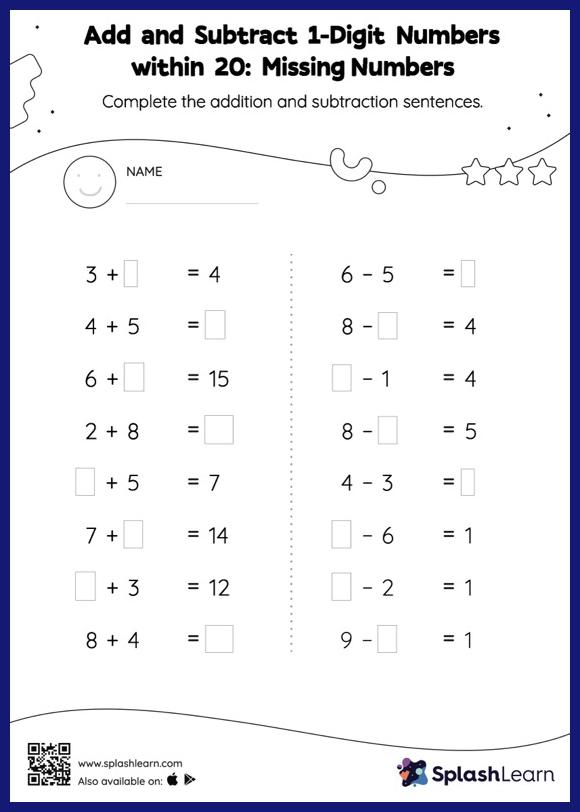# Add and Subtract 1-Digit Numbers within 20: Missing Numbers Worksheet

Home > Add and Subtract 1-Digit Numbers within 20: Missing NumbersThis add and subtract 1-digit numbers within 20 worksheet consists of tasks to help your young mathematician develop fluency with addition and subtraction. To find the missing number on this add and subtract 1-digit numbers within 20 worksheet, students can utilize the count-on strategy or the connection between addition and subtraction.